Anúncio

# 14. Recursion.pdf

21 de Nov de 2022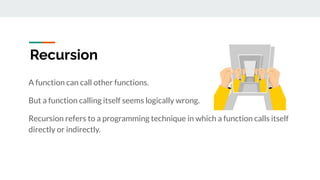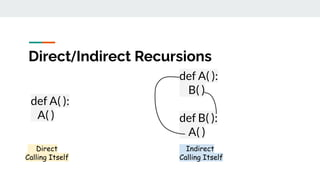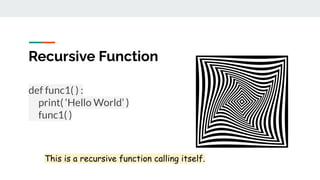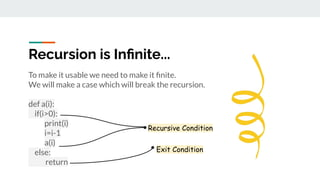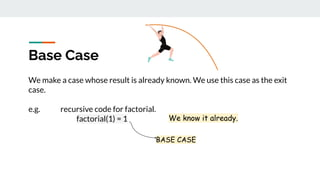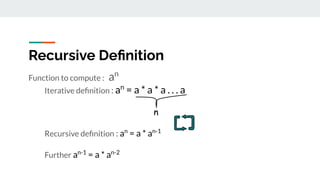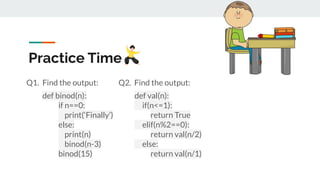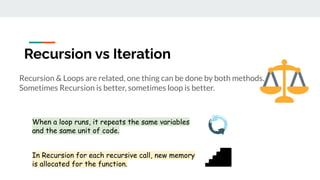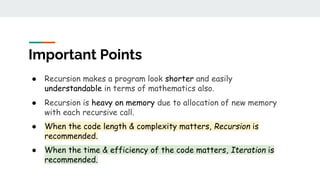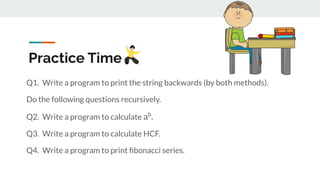1 de 12
Anúncio

### 14. Recursion.pdf

1. Recursion Intro, Recursive functions Recursion vs Iteration -> By Apni Kaksha <-
2. Recursion A function can call other functions. But a function calling itself seems logically wrong. Recursion refers to a programming technique in which a function calls itself directly or indirectly.
3. Direct/Indirect Recursions def A( ): A( ) def A( ): B( ) def B( ): A( ) Direct Calling Itself Indirect Calling Itself
4. Recursive Function def func1( ) : print( ‘Hello World’ ) func1( ) This is a recursive function calling itself.
5. Recursion is Inﬁnite... To make it usable we need to make it ﬁnite. We will make a case which will break the recursion. def a(i): if(i>0): print(i) i=i-1 a(i) else: return Recursive Condition Exit Condition
6. Base Case We make a case whose result is already known. We use this case as the exit case. e.g. recursive code for factorial. factorial(1) = 1 We know it already. BASE CASE
7. Function to compute : an Iterative deﬁnition : an = a * a * a . . . a n Recursive deﬁnition : an = a * an-1 Further an-1 = a * an-2 Recursive Deﬁnition
8. Practice Time Q1. Find the output: def binod(n): if n==0: print(‘Finally’) else: print(n) binod(n-3) binod(15) Q2. Find the output: def val(n): if(n<=1): return True elif(n%2==0): return val(n/2) else: return val(n/1)
9. Recursion & Loops are related, one thing can be done by both methods. Sometimes Recursion is better, sometimes loop is better. Recursion vs Iteration When a loop runs, it repeats the same variables and the same unit of code. In Recursion for each recursive call, new memory is allocated for the function.
10. ● Recursion makes a program look shorter and easily understandable in terms of mathematics also. ● Recursion is heavy on memory due to allocation of new memory with each recursive call. ● When the code length & complexity matters, Recursion is recommended. ● When the time & efficiency of the code matters, Iteration is recommended. Important Points
11. Practice Time Q1. Write a program to print the string backwards (by both methods). Do the following questions recursively. Q2. Write a program to calculate ab . Q3. Write a program to calculate HCF. Q4. Write a program to print ﬁbonacci series.
Anúncio